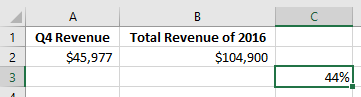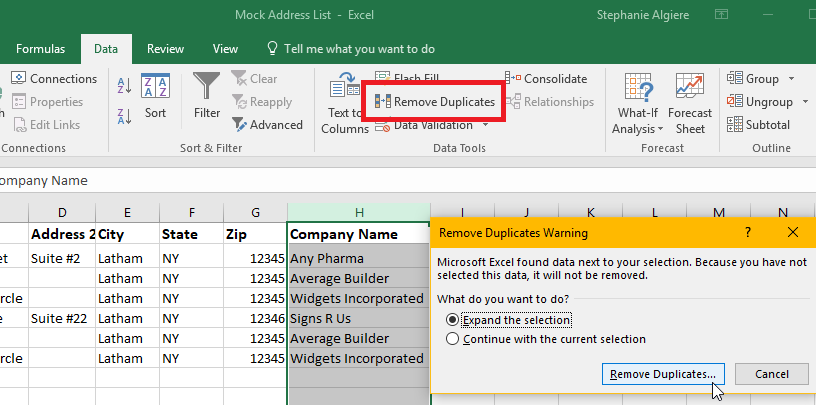# Tip of the Week: How To Use Excel To Its Full Potential## Tip of the Week: How To Use Excel To Its Full PotentialMicrosoft Office is arguably the most popular document suite in the world. Businesses of all sizes use them extensively in their daily operations. In fact, many employers require potential team members have advanced knowledge of various Office applications in order to qualify for a vacant position. Still, despite being so widely used, only a fraction of users are aware of the application’s true capabilities or which features and formulas they could use to make their daily tasks and processes easier to manage.

Before we started with formulas, here’s a bit of a refresher on where to enter the formulas and how they’re formatted. Followed by a few helpful tips and tricks to use in Excel that will scale back your work even further!

Entering and Formatting Formulas
Using formulas in Excel is not nearly as scary as one might think. The following breakdown details the basic components of an Excel formula, as well as a generalized-process that users can apply to the majority of the formulas.

• In order to indicate to Excel that you’re going to be entering a formula, you’ll need to enter an ‘=’ in the formula bar before adding in the equation you’re going to use.
• Find and enter the appropriate function. If you’re not sure which action applies, access the formula tab and search for your desired function.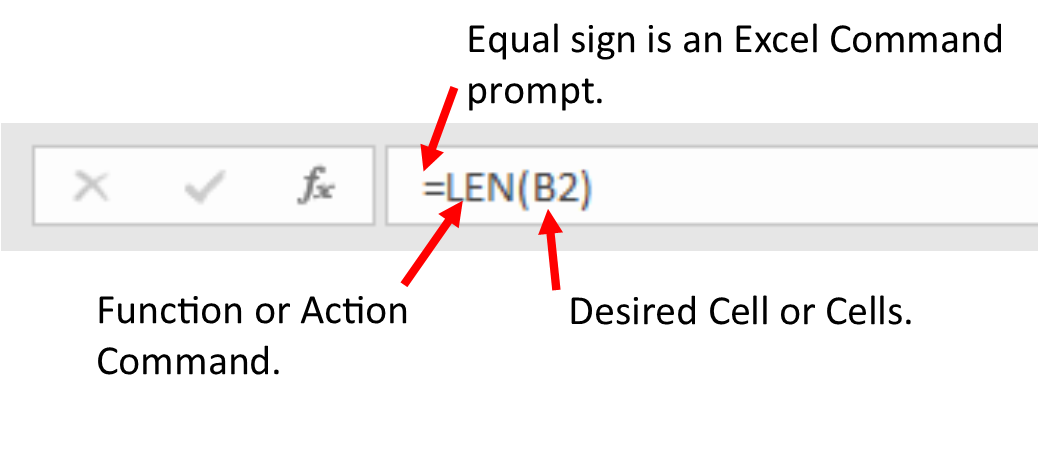• Next, add the letter and number for each of the cells you want to be involved with function inside the parenthesis.
• Once your formula is complete, hit the ‘Enter’ key and, if entered correctly, your data will be automatically calculated and updated.

Here’s three formulas that you can put to use when working in Excel!

Measure Characters in a Cell

• Formula: =len(cell)
• Function: This action will count the number of letters, numbers and characters that are in the cell you have entered into the formula.
• Example: Twitter has a character cap of 140, not including the links. To organize and schedule several twitter posts ahead of time, use a spreadsheet to make sure the posts are 140 characters or less before uploading them.

Calculate a Sum

• Formula: =sum(cell1:cell2)
• Function: This action will total a group of series cells. The colon tells Excel to calculate all the cells between the first cell and second in the formula.
• Example: =sum(a1:a12)
Choosing cell A1 for the first cell and A12 for the second one will automatically calculate the sum of all the numbers in Column A, cells 1 through 12.

Calculate Percentage

• Formula: =(cell1/cell2)
• Function: This action will automatically calculate the percentage. It’s critical for each function within the formula.
1.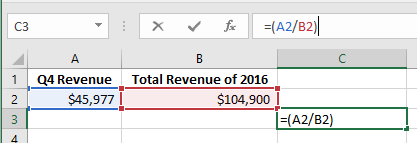2.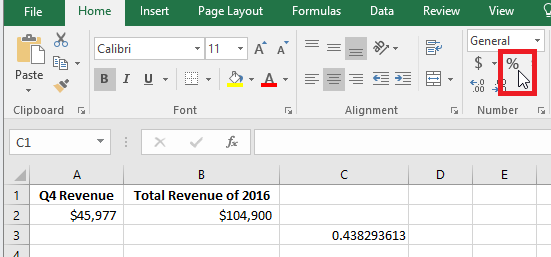3.